3.1. Goto-Nambu Strings

Our aim now is to introduce extra fields into the problem. The simple Lagrangian we saw in previous sections was a good approximation for ideal structureless strings, known under the name of Goto-Nambu strings [Goto, 1971; Nambu, 1970]. Additional fields coupled with the string-forming Higgs field often lead to interesting effects in the form of generalized currents flowing along the string core.

But before taking into full consideration the internal structure of strings we will start by setting the scene with the simple Abelian Higgs model (which describes scalar electrodynamics) in order to fix the notation etc. This is a prototype of gauge field theory with spontaneous symmetry breaking G = U(1){1}. The Lagrangian reads [Higgs, 1964]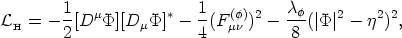(17)

with gauge covariant derivative Dµ =µ + i q A(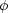)µ, antisymmetric tensor F()µ=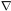µ A()-A()µ for the gauge vector field A(), and complex scalar field= ||eiwith gauge coupling q.

The first solutions for this theory were found by Nielsen & Olesen . A couple of relevant properties are noteworthy:

• the mass per unit length for the string is µ = U ~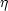2. For GUT local strings this gives µ ~ 1022g / cm, while one finds µ ~2 ln(r / ms-1)if strings are global, due to the absence of compensating gauge fields. This divergence is in general not an issue, because global strings only in few instances are isolated; in a string network, a natural cutoff is the distance to the neighboring string.
• There are essentially two characteristic mass scales (or inverse length scales) in the problem: ms ~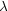1/2and mv ~ q, corresponding to the inverse of the Compton wavelengths of the scalar (Higgs) and vector (A()) particles, respectively.
• There exists a sort of screening of the energy, called `Higgs screening', implying a finite energy configuration, thanks to the way in which the vector field behaves far from the string core: A(1 / qr) d/ dfor r.

After a closed path around the vortex one has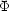(2) =(0), which implies that the winding phaseshould be an integer times the cylindrical angle, namely= n. This integer n is dubbed the `winding number'. In turn, from this fact it follows that there exists a tube of quantized `magnetic' flux, given by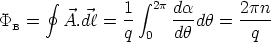(18)

In the string there is a sort of competing effect between the fields: the gauge field acts in a repulsive manner; the flux doesn't like to be confined to the core and B lines repel each other. On the other hand, the scalar field behaves in an attractive way; it tries to minimize the area where V()0, that is, where the field departs from the true vacuum.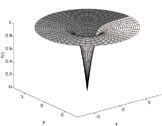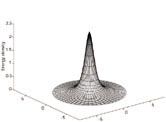Figure 1.6 Higgs field and energy profiles for Goto-Nambu cosmic strings. The left panel shows the amplitude of the Higgs field around the string. The field vanishes at the origin (the false vacuum) and attains its asymptotic value (normalized to unity in the figure) far away from the origin. The phase of the scalar field (changing from 0 to 2) is shown by the shading of the surface. In the right panel we show the energy density of the configuration. The maximum value is reached at the origin, exactly where the Higgs is placed in the false vacuum. [Hindmarsh & Kibble, 1995].

Finally, we can mention a few condensed-matter `cousins' of Goto-Nambu strings: flux tubes in superconductors [Abrikosov, 1957] for the nonrelativistic version of gauge strings (corresponds to the Cooper pair wave function). Also, vortices in superfluids, for the nonrelativistic version of global strings (corresponds to the Bose condensate wave function). Moreover, the only two relevant scales of the problem we mentioned above are the Higgs mass ms and the gauge vector mass mv. Their inverse give an idea of the characteristic scales on which the fields acquire their asymptotic solutions far away from the string `location'. In fact, the relevant core widths of the string are given by ms-1 and mv-1. It is the comparison of these scales that draws the dividing line between two qualitatively different types of solutions. If we define the parameter= (ms / mv)2, superconductivity theory says that< 1 corresponds to Type I behavior while> 1 corresponds to Type II. For us,< 1 implies that the characteristic scale for the vector field is smaller than that for the Higgs field and so magnetic field B flux lines are well confined in the core; eventually, an n-vortex string with high winding number n stays stable. On the contrary,> 1 says that the characteristic scale for the vector field exceeds that for the scalar field and thus B flux lines are not confined; the n-vortex string will eventually split into n vortices of flux 2/q. In summary: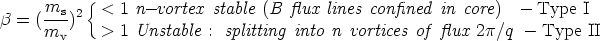(19)